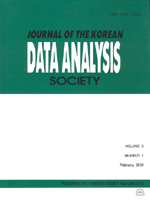상세검색
최근 검색어 전체 삭제
다국어입력
즐겨찾기0KCI등재 학술저널

# Nonparametric c-sample Test for Ordered Alternatives on Ranked-set Samples

• 등재여부 : KCI등재
• 1999.12
• 99 - 109 (11 pages)

In this paper, we consider c -sample nonparametric testing problem for ordered alternatives on ranked-set samples(RSS) and obtain the asymptotic relative efficiencies of the proposed test statistic with respect to Jonckheere type test statistic and statistic U based on simple random sampling(SRS). From the simulation work, we compare the empirical power of the proposed test statistic with Jonckheere type test statistic and statistic U based on SRS, where the underlying distributions are uniform, normal, double exponential, logistic and Cauchy distribution.

1. Introduction

2. Test Statistic

3. Asymptotic Properties

4. Power Comparison and Conclusions

References